﻿ 基于12A′基态势能面的H + DBr (v = 0, j = 0)反应几率的理论研究 Theoretical Study of the Probabilities for the Reaction H + DBr (v = 0, j = 0) on the 12A' Potential Energy

Applied Physics
Vol. 09  No. 05 ( 2019 ), Article ID: 30200 , 7 pages
10.12677/APP.2019.95027

Theoretical Study of the Probabilities for the Reaction H + DBr (v = 0, j = 0) on the 12A' Potential Energy Surface

Yuliang Wang*, Hui Li, Dezhi Su, Mingyu Zhou

School of Basic Sciences for Aviation, Naval Aviation University, Yantai Shandong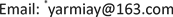Received: Apr. 25th, 2019; accepted: May 7th, 2019; published: May 14th, 2019ABSTRACT

Quasi-classical trajectory calculations were carried out for H + DBr (v = 0, j = 0) over the collision energy range 0 - 2.0 eV on the 12A' potential energy surface. The probabilities for both the exchange and abstraction channels of the reaction at total angular momentum J = 0, 10, 20, 30, 40 are predicted in this work. The attack angle dependent probabilities of H + DBr at J = 0 are calculated. It was found that the influence of attack angle on the distribution of probabilities for both two channels is significant because of the different effective potential barrier heights at different attack angles.

Keywords:H + DBr Reaction, Attack Angle, Reaction Probability, Reaction Dynamics1. 引言

2. 计算理论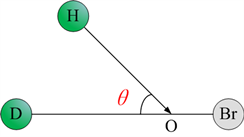Figure 1. The definition figure of the attack angle

3. 结果与讨论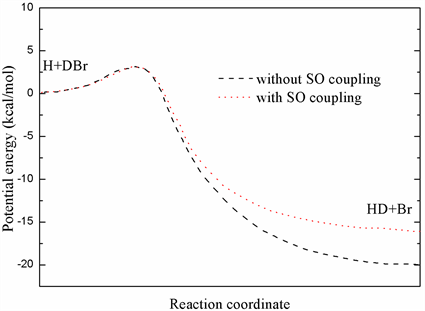Figure 2. Minimum energy pathways of abstraction for the collinear configuration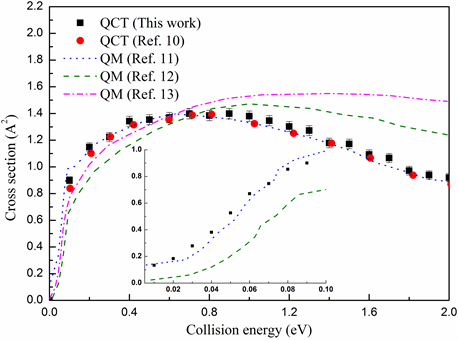Figure 3. Comparison of integral cross section for abstraction between present work and other theoretical results in the text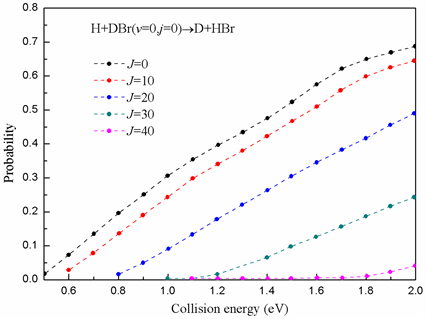Figure 4. The reaction probabilities for exchange channel at different J values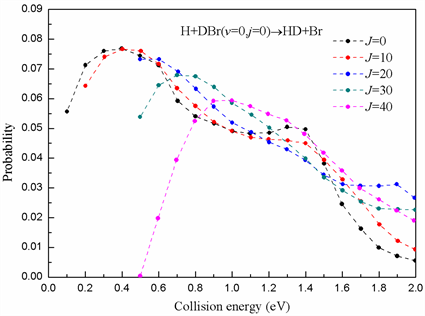Figure 5. The reaction probabilities for abstraction channel at different J valuesFigure 6. The attack angle dependent probability of exchange channel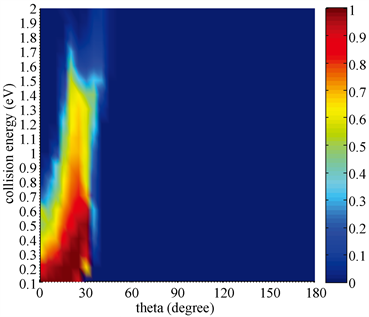Figure 7. The attack angle dependent probability of abstraction channel

4. 结论

Theoretical Study of the Probabilities for the Reaction H + DBr (v = 0, j = 0) on the 12A' Potential Energy[J]. 应用物理, 2019, 09(05): 226-232. https://doi.org/10.12677/APP.2019.95027

1. 1. Aker, P.M., Germann, G.J. and Valentini, J.J. (1989) State-to-State Dynamics of H+HX Collisions. I. The H+HX→H2+X (X=Cl, Br, I) Abstraction Reactions at 1.6 eV Collision Energy. Journal of Chemical Physics, 90, 4795-4808. https://doi.org/10.1063/1.456574

2. 2. Pomerantz, A.E., Camden, J.P., Chiou, A.S., Ausfelder, F., Chawla, N., Hase, W.L. and Zare, R.N. (2005) Reaction Products with Internal Energy beyond the Kinematic Limit Result from Trajectories Far from the Minimum Energy Path: An Example from H+HBrH2 + Br. Journal of American Society, 127, 16368-16369. https://doi.org/10.1021/ja055440a

3. 3. Zhang, J.Y., Jankunas, J., Bartlett, N.C.M., Goldberg, N.T. and Zare, R.N. (2010) Search for Br Production in the D + DBr Reaction. Journal of Chemical Physics, 132, 084301. https://doi.org/10.1063/1.3319717

4. 4. Lynch, G.C., Truhlar, D.G., Brown, F.B. and Zhao, J.G. (1995) A New Potential Energy Surface for H2Br and Its Use to Calculate Branching Ratios and Kinetic Isotope Effects for the H + HBr Reaction. Journal of Chemical Physics, 99, 207-225. https://doi.org/10.1021/j100001a034

5. 5. Mielke, S.L., Tawa, G.J., Truhlar, D.G. and Schwenke, D.W. (1995) Quantum Photochemistry. Accurate Quantum Scattering Calculations for an Electronically Nonadiabatic Reaction. Chemical Physics Letters, 234, 57-63. https://doi.org/10.1016/0009-2614(94)01515-w

6. 6. Kurosaki, Y. and Takayanagi, T. (2003) Global ab initio Potential Energy Surfaces for the Lowest Three Doublet States (12A′, 22A′, and 12A′′) of the BrH2 System. Journal of Chemical Physics, 119, 7838-7856. https://doi.org/10.1063/1.1609398

7. 7. Kurosaki, Y. and Takayanagi, T. (2005) A Modified Version of the Ana-lytical Potential Function for the Global ab initio Ground-State Potential Energy Surface of the BrH2 System. Chemical Physics Letters, 406, 121-125. https://doi.org/10.1016/j.cplett.2005.02.106

8. 8. Jiang, B., Xie, C.J. and Xie, D.Q. (2011) New ab initio Potential Energy Surface for BrH2 and Rate Constants for the H + HBrH2 + Br Abstraction Reaction. Journal of Chemical Physics, 134, Article ID: 114301. https://doi.org/10.1063/1.3563750

9. 9. Quan, W.L., Song, Q. and Tang, B.Y. (2007) The Effects of the Rotational Excitation on the Br+H2 Reaction and Its Dependence on the Potential Energy Surfaces. Chemical Physics Letters, 442, 228-232. https://doi.org/10.1016/j.cplett.2007.05.105

10. 10. Zhang, W.Q., Cong, S.L., Zhang, C.H., Xu, X.S. and Chen, M.D. (2009) Theoretical Study of Dynamics for the Abstraction Reaction H′ + HBr (v=0, j=0)H′H + Br. The Journal of Physical Chemistry A, 113, 4192-4197. https://doi.org/10.1021/jp8105716

11. 11. Fu, B.N. and Zhang, D.H. (2007) A Time-Dependent Quantum Dynamical Study of the H + HBr Reaction. The Journal of Physical Chemistry A, 111, 9516-9521. https://doi.org/10.1021/jp073811z

12. 12. Xie, C.J., Jiang, B. and Xie, D.Q. (2011) State-to-State Quantum Dynamics of the H + HBr Reaction: Competition between the Abstraction and Exchange Reactions, Journal of Chemical Physics, 134, Article ID: 184303. https://doi.org/10.1063/1.3589407

13. 13. Zhang, A.J., Zhang, P.Y., Chu, T.S., Han, K.L. and He, G.Z. (2012) Quantum Dynamical Study of the Electronic Nonadiabaticity in the D + DBr→Br(Br*) + D2 Reaction on New Diabatic Potential Energy Surfaces. Journal of Chemical Physics, 137, Article ID: 194305. https://doi.org/10.1063/1.4766355

NOTES

*通讯作者。